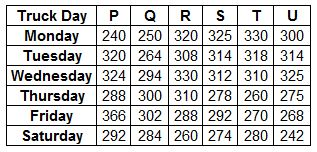### SBI Associate PO 2010 Question 142

Instructions

Study the table carefully to answer the question that follow:
Distance (in kms) travelled by six trucks on six different days of the weekQuestion 142

# If the speed of truck P on Monday was 19.2 kmph, what was the time taken by it to cover the given distance?

Solution

Distance travelled by P on Monday = 240 km
Speed = 19.2 kmph
Speed = Distance/Time
Time = $$\frac{240}{19.2} = 12.5$$ hr
$$\therefore$$ P took 12 hr 30 minutes to cover the distance.